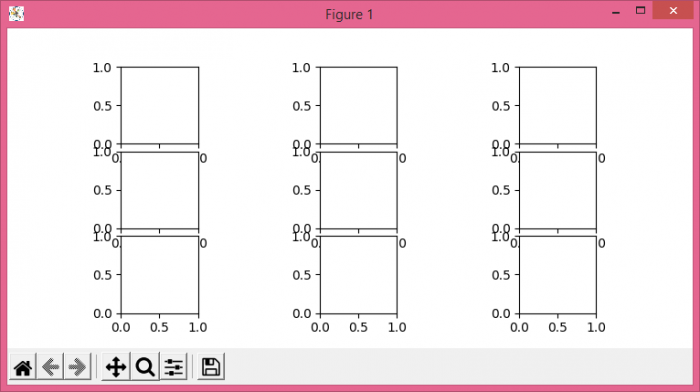# How to remove the space between subplots in Matplotlib.pyplot?

To remove the space between subplots in matplotlib, we can use GridSpec(3, 3) class and add axes as a subplot arrangement.

## Steps

• Set the figure size and adjust the padding between and around the subplots.
• Add a grid layout to place subplots within a figure.
• Update the subplot parameters of the grid
• Iterate in the range of dimension of grid specs.
• Add a subplot to the current figure.
• Set the aspect ratios.
• To display the figure, use show() method.

## Example

import matplotlib.pyplot as plt
import matplotlib.gridspec as gridspec
plt.rcParams["figure.figsize"] = [7.50, 3.50]
plt.rcParams["figure.autolayout"] = True
gs1 = gridspec.GridSpec(3, 3)
gs1.update(wspace=0.5, hspace=0.1)
for i in range(9):
ax1 = plt.subplot(gs1[i])
ax1.set_aspect('equal')
plt.show()

## Output